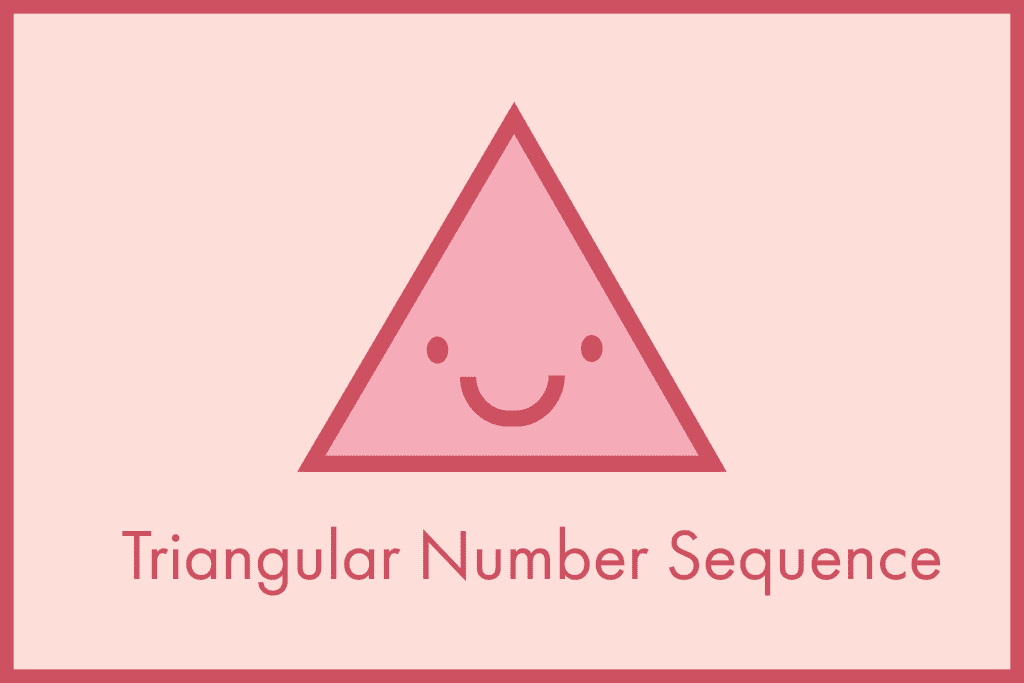How Cheenta works to ensure student success?
Explore the Back-Story

# Triangular Number Sequence | Explanation with Application## Triangular Number Sequence: Definition

In Triangular Number Sequence, the numbers are in the form of an equilateral triangle arranged in a series or sequence. These numbers are in the sequence of 1,3,6,10,15,21 and so on. In this representation, the numbers are represented by dots. So using the sequence notation we can write {1,3,6,10,15,21,28,............}.

### Something More ..............

As in definition, it is mentioned that these numbers are represented using equilateral triangles with dots. So we will start with a single dot at first then there will be a triangle and in each vertex, there will be one dot so total 3 dots will be there and then there will be a triangle with 3 dots at vertices and three dots in the middle of each side of the triangle and so on.

## Let's see with a picture :

Thus we can understand what I mentioned above .

1)The very first picture only one dot is there,

2)In 2nd picture a row is added with 2 dots to the 1st picture,

3)in 3rd picture a row is added with 3 dots to the 2nd picture and so on ........

So the pattern is , 1 -> 1+2 --> 1+2+3 ---> 1+2+3+4 ----> 1+2+3+4+5 -----> ..........................

If we rearrange all the dots :

Now doubling each number of dots in each representation we get :

Now depending on the number dots in rows and columns in each presentation we can easily generate the general formula for this sequence using " n " where " n " represents the number of dots we are using in each presentation.

The formula can be : $T _{n} = \frac {n \times (n+1)}{2}$

As we have taken each number twice so to generate the formula for the actual Triangular Number Sequence we have to divide it by 2.

## Some Application of Triangular Number Sequence:

Find the 10th term in the triangular number sequence.

Here our generated formula will work

$T_{10} = \frac {10 \times (10+1)}{2}$

$T_{10} = \frac {10 \times 11}{2}$

$T_{10} = \frac {110}{2}$

$T_{10} = 55$ (Answer )

Find the 99 th term in triangular number sequence .

$T_{99} = \frac {99 \times (99+1)}{2}$

$T_{99} = \frac {99 \times 100}{2}$

$T_{99} = 99 \times 50$

$T_{99} = 4950$ (Answer)

## Triangular Number Sequence: Definition

In Triangular Number Sequence, the numbers are in the form of an equilateral triangle arranged in a series or sequence. These numbers are in the sequence of 1,3,6,10,15,21 and so on. In this representation, the numbers are represented by dots. So using the sequence notation we can write {1,3,6,10,15,21,28,............}.

### Something More ..............

As in definition, it is mentioned that these numbers are represented using equilateral triangles with dots. So we will start with a single dot at first then there will be a triangle and in each vertex, there will be one dot so total 3 dots will be there and then there will be a triangle with 3 dots at vertices and three dots in the middle of each side of the triangle and so on.

## Let's see with a picture :

Thus we can understand what I mentioned above .

1)The very first picture only one dot is there,

2)In 2nd picture a row is added with 2 dots to the 1st picture,

3)in 3rd picture a row is added with 3 dots to the 2nd picture and so on ........

So the pattern is , 1 -> 1+2 --> 1+2+3 ---> 1+2+3+4 ----> 1+2+3+4+5 -----> ..........................

If we rearrange all the dots :

Now doubling each number of dots in each representation we get :

Now depending on the number dots in rows and columns in each presentation we can easily generate the general formula for this sequence using " n " where " n " represents the number of dots we are using in each presentation.

The formula can be : $T _{n} = \frac {n \times (n+1)}{2}$

As we have taken each number twice so to generate the formula for the actual Triangular Number Sequence we have to divide it by 2.

## Some Application of Triangular Number Sequence:

Find the 10th term in the triangular number sequence.

Here our generated formula will work

$T_{10} = \frac {10 \times (10+1)}{2}$

$T_{10} = \frac {10 \times 11}{2}$

$T_{10} = \frac {110}{2}$

$T_{10} = 55$ (Answer )

Find the 99 th term in triangular number sequence .

$T_{99} = \frac {99 \times (99+1)}{2}$

$T_{99} = \frac {99 \times 100}{2}$

$T_{99} = 99 \times 50$

$T_{99} = 4950$ (Answer)

## Subscribe to Cheenta at Youtube

This site uses Akismet to reduce spam. Learn how your comment data is processed.

### Knowledge Partner